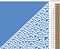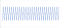# More patterns in cellular automata

I’ve been recently obsessed with Cellular Automata, specifically elementary CA. I stumbled on these while playing around with entropy in random noise (an odd and futile hobby, I know), as some of the transforms I was applying turned out to be identical to some of the elementary cellular automata rules.

Typically, each subsequent generation of a cellular automaton is derived from the repeated application of a single rule, like the famous Rule 30:

While Rule 30 is well studied for its apparently chaotic and random nature, I wanted to see if there were any meaningful patterns in the relationships between each subsequent generation.

Here’s 3 such explorations.

Given any generation of any rule, such as Rule 30, what other rules could generate the next row of output? Are there any patterns in these rules?

I have written a very quick script that, given a starting rule and a number of iterations, will output which alternative rules will output the next generation identically.

This could be a useful tool for spotting patterns in various rules, for example Rule 90 seems to have a fair number of equivalent rules, alternating on odd and even rows, with generations 1, 3, 7, 15, 31, 63 etc having their own special set of equivalent rules (or line 2, 4, 8, 16, 32, 64 etc if the initial row “1” is counted — though not technically a “generation”)

This script is a useful tool for finding patterns in any of the 256 rules. Finding patterns would be the first step to figuring out if they have any kind of special significance.

Rule 30’s first handful of generations can be produced by alternating between rules 62 and 27 (among others), before its values become unique to Rule 30 (at least for the first 2000 iterations I tested locally):

For at least the first 10 iterations (generations 0 to 9) The rules for odd and even rows seems generally mutually exclusive.

This fiddle rotates an array of rules for each subsequent generation, in this example 62 and 27:

Does this pattern hold true in general or is it just a coincidence?

I generated a range of rows from an arbitrary input to rule 30.

The images below show Odd and Even rows from this output:

Taking the sum of odd and even cells allows us to visualise similarities and differences.

Values of 1 show where the even and odd rows are different (e.g. even XOR odd), while values of 2 and 0 show where the even and odd rows are the same.

If we calculate just the difference (XOR) of even and odd rows, they are on average slightly more than 50% different to one another.

Is the difference between odd and even rows significant? What about the difference between subsequent rows on;y?

Looking at the difference between the decimal values of each generation of Rule 30 (this time seeded with a single 1):Decimal representation of Rule 30 generations, highlighting the difference (n / n-1) / 4 — (dividing by 4 counters the factor of 4 increase caused by length of the binary sequence increasing by 2 each generation)

Graphing the difference between generations / 4 — we see the difference factor alternates between <1 and >1 for even and odd rows:Interestingly, the trendline at least in the first ~40 iterations increases >1, does it continue to increase or does it oscillate?

Looking at Rule 30 from 3 different angles; (1) the patterns from analysing the application of different rules per generation, (2) the difference of even and odd rows, and (3) the difference between the decimal value of each row strongly indicates a Modulus of 2 would be involved in any function to simplify the calculation of arbitrary output for any given row of Rule 30 — if such a function exists.

Understanding why the patterns between even and odd rules break down in example (1) could yield further understanding, examining patterns in the differences and similarities of even and odd rows in (2) could be another avenue of inquiry, as could exploring the trends and patterns in the difference in decimal representations from example (3).

I have another post coming soon on some other interesting patterns I’ve found. While I continue to stumble around I am well aware this is a well researched puzzle, so if these patterns are already well known I would love some pointers from experts so that I am not reinventing the wheel, in addition to any general feedback and ideas.